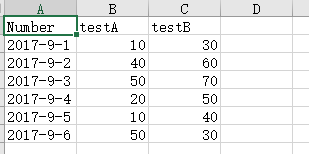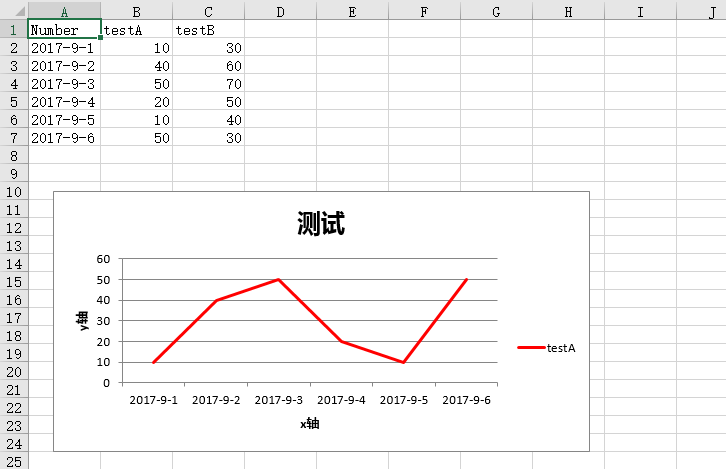• 2020-11-24 23:13:30

1、xlsxwriter模块的简单使用：

xlsxwriter模块主要用来生成excel表格，插入数据、插入图标等表格操作。

1.1 基本功能

import xlsxwriter #导入模块

workbook = xlsxwriter.Workbook('new_excel.xlsx') #新建excel表

data = [

['2017-9-1','2017-9-2','2017-9-3','2017-9-4','2017-9-5','2017-9-6'],

[10,40,50,20,10,50],

[30,60,70,50,40,30],

] #自己造的数据

worksheet.write_column('A2',data)

worksheet.write_column('B2',data)

worksheet.write_column('C2',data) #将数据插入到表格中

workbook.close() #将excel文件保存关闭，如果没有这一行运行代码会报错

查看生成excel的结果：1.2 将excel中插入折线图1 import xlsxwriter #导入模块2

3 workbook = xlsxwriter.Workbook('new_excel.xlsx') #创建新的excel4

9 data =[10 ['2017-9-1','2017-9-2','2017-9-3','2017-9-4','2017-9-5','2017-9-6'],11 [10,40,50,20,10,50],12 [30,60,70,50,40,30],13 ] #自己造的数据14

17 worksheet.write_column('A2',data)18 worksheet.write_column('B2',data)19 worksheet.write_column('C2',data) #将数据插入到表格中20

21 chart_col = workbook.add_chart({'type':'line'}) #新建图表格式 line为折线图22 chart_col.add_series( #给图表设置格式，填充内容23 {24 'name':'=sheet1!$B$1',25 'categories':'=sheet1!$A$2:$A$7',26 'values': '=sheet1!$B$2:$B$7',27 'line': {'color': 'red'},28 }29 )30

31 chart_col.set_title({'name':'测试'})32 chart_col.set_x_axis({'name':"x轴"})33 chart_col.set_y_axis({'name':'y轴'}) #设置图表表头及坐标轴34

35 chart_col.set_style(1)36

37 worksheet.insert_chart('A10',chart_col,{'x_offset':25,'y_offset':10}) #放置图表位置38

39 workbook.close()

View Code

生成图表如下图2、xlsxwriter模块常用功能介绍：

2.1、设置单元格的格式：

2.1.1、通过字典的方式直接设置格式。

1 workfomat =workbook.add_format({2 'bold': True, #字体加粗3 'border':1, #单元格边框宽度4 'align': 'center', #对齐方式5 'valign': 'vcenter', #字体对齐方式6 'fg_color': '#F4B084', #单元格背景颜色7 })

2.1.2、通过format对象的方式设置单元格格式。

3 workfomat.set_num_format('0.00') #格式化数据格式为小数点后两位

4 workfomat.set_align('center') #设置对齐方式

5 workfomat.set_fg_color('blue') #设置单元格背景颜色

6 workfomat.set_bg_color('red') #设置单元格背景颜色 (经测试和上边的功能一样)

2.1.3、一些单元表的操作，像这样的操作还有好多，可以根据自己的需要去进行研究。

1 worksheet.merge_range('D1:D7','合并单元格') #合并单元格

2 worksheet.set_tab_color('red') #设置sheet标签颜色

3 worksheet.set_column('A:D',25) #设置A到D列的列宽为25

4 worksheet.write_formula('E2','=B2/C2') #设置表格中的计算，‘E2’是计算结果，'=B2/C2'是计算公式

2.2、常用图表类型：

1 #area：面积图

2 #bar：直方图

3 #colume：柱状图

4 #line：折线图

5 #pie：饼图

6 #doughnut：环形图

7 #sactter：散点图

8 #stock：股票趋势图

更多相关内容
• matplotlib 是python最著名的绘图库，它提供了一整套和matlab相似的命令API，十分适合交互式地行制图。而且也可以方便地将它作为绘图控件，嵌入GUI应用程序中。它的文档相当完备，并且Gallery页面中有上百幅缩略，...

matplotlib 是python最著名的绘图库，它提供了一整套和matlab相似的命令API，十分适合交互式地行制图。而且也可以方便地将它作为绘图控件，嵌入GUI应用程序中。

它的文档相当完备，并且Gallery页面中有上百幅缩略图，打开之后都有源程序。因此如果你需要绘制某种类型的图，只需要在这个页面中浏览/复制/粘贴一下，基本上都能搞定。

在Linux下比较著名的数据图工具还有gnuplot，这个是免费的，Python有一个包可以调用gnuplot，但是语法比较不习惯，而且画图质量不高。

Matplotlib则比较强：Matlab的语法、python语言、latex的画图质量(还可以使用内嵌的latex引擎绘制的数学公式)。

matplotlib绘制折线图

1. line chart

import numpy as np

import matplotlib.pyplot as plt

x = np.linspace(0, 2 * np.pi, 100)

y1, y2 = np.sin(x), np.cos(x)

plt.plot(x, y1)

plt.plot(x, y2)

plt.title('line chart')

plt.xlabel('x')

plt.ylabel('y')

plt.show()2. 图例

在plot的时候指定label，然后调用legend方法可以绘制图例。例如：

import numpy as np

import matplotlib.pyplot as plt

x = np.linspace(0, 2 * np.pi, 100)

y1, y2 = np.sin(x), np.cos(x)

plt.plot(x, y1, label='y = sin(x)')

plt.plot(x, y2, label='y = cos(x)')

plt.legend()

plt.show()legend方法可接受一个loc关键字参数来设定图例的位置，可取值为数字或字符串：

0: ‘best'

1: ‘upper right'

2: ‘upper left'

3: ‘lower left'

4: ‘lower right'

5: ‘right'

6: ‘center left'

7: ‘center right'

8: ‘lower center'

9: ‘upper center'

10: ‘center'

3. 线的样式

(1)颜色

plot方法的关键字参数color(或c)用来设置线的颜色。可取值为：

1、颜色名称或简写

b: blue

g: green

r: red

c: cyan

m: magenta

y: yellow

k: black

w: white

2、#rrggbb

3、(r, g, b) 或 (r, g, b, a)，其中 r g b a 取均为[0, 1]之间

4、[0, 1]之间的浮点数的字符串形式，表示灰度值。0表示黑色，1表示白色

(2)样式

plot方法的关键字参数linestyle(或ls)用来设置线的样式。可取值为：

-, solid

--, dashed

-., dashdot

:, dotted

'', ' ', None

(3)粗细

设置plot方法的关键字参数linewidth(或lw)可以改变线的粗细，其值为浮点数。4. marker

以下关键字参数可以用来设置marker的样式：

marker

markeredgecolor 或 mec

markeredgewidth 或 mew

markerfacecolor 或 mfc

markerfacecoloralt 或 mfcalt

markersize 或

ms

其中marker可取值为：

'.': point

marker

',': pixel

marker

'o': circle

marker

'v':

triangle_down marker

'^':

triangle_up marker

'

'>': triangle_right marker

'1':

tri_down marker

'2': tri_up

marker

'3':

tri_left marker

'4':

tri_right marker

's': square

marker

'p':

pentagon marker

'*': star

marker

'h':

hexagon1 marker

'H':

hexagon2 marker

' ': plus

marker

'x': x

marker

'D': diamond

marker

'd':

thin_diamond marker

'|': vline

marker

'_': hline

marker

例如： import numpy as np

import matplotlib.pyplot as plt

x = np.linspace(0, 2 * np.pi, 10)

y1, y2 = np.sin(x), np.cos(x)

plt.plot(x, y1, marker='o', mec='r', mfc='w')

plt.plot(x, y2, marker='*', ms=10)

plt.show()另外，marker关键字参数可以和color以及linestyle这两个关键字参数合并为一个字符串。例如：

import numpy as np

import matplotlib.pyplot as plt

x = np.linspace(0, 2 * np.pi, 10)

y1, y2 = np.sin(x), np.cos(x)

plt.plot(x, y1, 'ro-')

plt.plot(x, y2, 'g*:', ms=10)

plt.show()总结

以上就是这篇文章的全部内容了，希望本文的内容对大家的学习或者工作能带来一定的帮助

展开全文• geo（地理坐标系） geolines（地理坐标系线图） graph（关系图） heatmap（热力图）klinecandlestick（k线图） line（折线面积图） line3d（3d 折线图）liquid（水球图） map（地图） parallel（平行坐标系） pie...广告关闭

腾讯云11.11云上盛惠 ，精选热门产品助力上云，云服务器首年88元起，买的越多返的越多，最高返5000元！

import datetime import pandas_datareader.data as web df_stockload =web.datareader(600797.ss, yahoo, datetime.datetime(2018, 1, 1),datetime.datetime(2019, 1, 1))print(df_stockload.info()) # 替换 importmatplotlib.finance as mpf 画k线图import mpl_finance as mpf # 替换 importmatplotlib.finance as ...每次打印日志之后，翻日志再找其他k线工具来校对，这个过程简直泪流满面。 在无数次面对日志抓瞎以后，决心寻找一个好用的python k线工具，于是在知乎搜索 python k线，有很多不错的python k线案例：python如何绘制k线图？ https:www.zhihu.comquestion62611557 怎么样用python画k线图？ https:zhuanlan.zhihu...

- k线图：python画k线图的操作可以学很久，python里面画k线的包不是非常多，而且，处理x轴的日期不是非常容易，因为可以直接用的函数基本都是对应的自然日，但k线只画交易日，虽然交易日是连续的，但画出来会导致k线之间间隔不一样。 编程部分大概如上，python部分是这样，其他语言可以借鉴。 除此外，vba是比较特殊...

大数据文摘作品编译：大山、笪洁琼、yawei xia对于k线图，相信做交易的朋友都不陌生。 本文作者用简单明了的语言解释了三日k线的交易原则，也分享了如何用python绘制k线图的方法和代码。 关于日本k线交易据说日本人在十七世纪就已经运用技术分析的方法进行大米交易，一位名叫本间宗久的坂田大米贸易商发明了“蜡烛图...

对股票一窍不通的我也在此情此景进行了一波学习充电，股市的变化瞬息万千，有一种图可以用来清晰地反应一段时间内股市的变化情况，它就是k线图。 今天我们用python基于两种不同的方式来绘制k线图，它们都是python可视化的好帮手——matplotlib、pyecharts。 k线图这种图表源处于日本德川幕府时代，被当时日本米市的...

由于k线和成交量是两种类别的技术指标，我们不仅要将它们分别在两个子图上进行显示，而且需要协调两个子图的位置和比例。 此处导入matplotlib的gridspec模块创建子图，gridspec可自定义子图的位置和调整子图行和列的相对高度和宽度，如下所示：# 成交量可视化#绘制k线图+移动平均线+成交量 import numpy as npimport...df].plot(figsiz=(10,18))效果如下:? 用python炒股? k线图import matplotplib.pyplot as pltfrom matplotlib.daet importdateformatterfrom matplotlib.finance import date2num,candlestick_ohlcdef candleplot(data, title=):data = datalist = ].values]ax =plt.subplot()ax.set_title(title)ax.xaxis.set_major...先看用 matplotlib 画的 k 线图，密密麻麻啥都看不清楚，根本区分不了开盘价、最高价、最低价、收盘价。 再看用 pyecharts 画的 k 线图 (gif)，也是密...本文是 python 系列的第十篇python 入门篇 (上)python 入门篇 (下)数组计算之 numpy(上)数组计算之 numpy (下)科学计算之 scipy数据结构之 pandas (上)...

先看用 matplotlib 画的 k 线图，密密麻麻啥都看不清楚，根本区分不了开盘价、最高价、最低价、收盘价。 再看用 pyecharts 画的 k 线图 (gif)，也是密...得到了众多开发者的认可，而 python 就不用多说了。 当 python 遇到了 echarts，就变成了 pyecharts。 本帖需要的其他库如下：import numpy as npimport ...蜡烛图 即我们熟悉的k线图，通过开盘价，最高价，最低价，表示一个时间周期的交易情况，蜡烛图有许多的有意义图形，这里主要说几个我觉得有点道理的图形，长实体，十字星 长实体指单根k线的最高价与最低价相差很大，然后收盘价与开盘价分别非常靠近最高价最低价。 之所以这样是因为买方或者卖方非常强势。 可以用来...

该笔记源自尚学堂,非原创python 单词是“大蟒蛇”的意思。 但是龟叔不是喜欢蟒蛇才起这个名字，而是正在追剧：英国电视喜剧片《蒙提·派森的飞行马戏团》(monty python and the flying circus)。 使用 www.python.org 提供的 interactive shell 入门 python·特点可读性强可读性远比听上去重要的多得多。 一个程序会...

最近捣鼓ros的时候，发现github上有人用python实现了pid，虽然可能执行效率不高，但是用python写工具的时候还是很方便的。 从github上把代码搬下来，简单分析一下 ? 给代码: 在截个都看烦了的公式意思一下吧 ? #! usrbinpython## this file is part of ivpid.# copyright (c) 2015 ivmechmechatronics ltd. ## ivpid ...

表示处理过程输入输出框：表示数据输入或结果输出注释框：左边是虚线，右边是半框，不想画了流向线：表示程序执行路径连接点：表示多个流程图的连接方式 ...python3. 8.5下载地址：64位：https:www.python.orgftppython3.8. 5python-3. 8.5-amd64.exe32位：https:www.python.orgftppython3.8. 5python-3. 8.5.exe...画简单线图如下：#线图 s = series(np.random.randn(10).cumsum(),index=np.arange(0, 100, 10))s.plot()plt.show()显示： ? pandas.series.plot()的常见参数及说明如下：参数 说明 参数 说明 label 用于图例的标签 ax 要在其上进行绘制的matplotlibsubplot对象，如果没有设置，则使用当前matplotlib subplot style ...

由于公司的execl版本（v2010）偏低，没有画箱线图的功能，故我用python写了一小段程序，可以用来画箱线图。 绘图库使用的还是matplotlib。? 通过点击图形元素，可以很方便的修改总标题和轴标题，可以改变箱体的样式和颜色。 还可以添加水平参考线。? 源码如下主要由4个部分组成。 1. 实现绘图功能的mycanvas.pyfrom ...c.render_notebook() ? 6.4 k线图from pyecharts import options as optsfrom pyecharts.charts importkline data = , , , , , , , , , , , , , , , , , , , , , , , , , , , , , , ,k = ( kline() .add_xaxis() .add_yaxis(k线图, data)set_global_opts( yaxis_opts=opts.axisopts(is_scale=true),xaxis_opts=opts...geo（地理坐标系） geolines（地理坐标系线图） graph（关系图） heatmap（热力图）klinecandlestick（k线图） line（折线面积图） line3d（3d 折线图）liquid（水球图） map（地图） parallel（平行坐标系） pie（饼图）polar（极坐标系） radar（雷达图） sankey（桑基图） scatter（散点图）scatter3d（3d 散点图...python环境安装python资料分享群【 784758214 】群内有安装包和学习视频资料，零基础，进阶，领取永久的实战免费在线直播课程，大牛在线解答疑问。 希望可以帮助你快速了解python、学习python获取行情数据import pandas as pdimporttushare as ts通过股票代码获取股票数据,这里没有指定开始及结束日期df =ts.get_k...

（python2和3的教程可以选择一个深入学习）1. python3 简明教程2. python快速教程阶段3：web框架1. python django web框架2. python flask web框架阶段4：基础项目1. python 图片转字符画用 50 行 python 代码完成图片转字符画小工具。 将学习到 linux 命令行操作，python 基础，pillow 库的使用，argparse 库的使用 ...

展开全文• 折线图绘制

import numpy as np
import pandas as pd
import matplotlib.pyplot as mp
import matplotlib.pyplot as plt
plt.style.use(['science', 'no-latex'])

from matplotlib.pyplot import MultipleLocator
from matplotlib import rcParams
config = {
"font.family":'Times New Roman',  # 设置字体类型
"font.size": 4.5,
#     "mathtext.fontset":'stix',
}

rcParams.update(config)

import numpy as np
import pandas as pd
import matplotlib.pyplot as plt
plt.style.use(['science', 'no-latex'])
plt.rcParams['font.family'] = "Times New Roman"
x_index = [1,2,3,4,5,6,7,8,9,10]

# Data Prepare BEGIN
# Data located in
# E:/A_A_A_A_Papers/Federated_Learning/MU_base_on_IF/influence-release-master-Convex/influence-release-master/' \
#                'z_Retrain_on_model/Best_result/Experiment_data_save/save_params/save_params_mu.txt'
x = [200, 400, 600, 800, 1000, 1200, 1400, 1600, 1800, 2000]
y0_MNIST = []
y1_MNIST = []
y2_MNIST = []

y0_FASHION = []
y1_FASHION = []
y2_FASHION = []

y0_CIFAR10 = []
y1_CIFAR10 = []
y2_CIFAR10 = []

y0_SVHN = []
y1_SVHN = []
y2_SVHN = []

# Data Prepare END

# Plot BEGIN
fig = plt.figure(figsize=(4,3),dpi=300)

# @@@ Fig_1 BEGIN
ax0 = fig.add_subplot(2, 2 , 1)
ax0.set(ylabel='Accuracy $D_{test}$')
ax0.set(xlabel='Forgotten Samples')

x_major_locator=MultipleLocator(200)
ax0.xaxis.set_major_locator(x_major_locator)

ax0.tick_params(axis='x', which='both',colors='black', direction='out')

ax0.tick_params(axis='y', colors='dodgerblue')
ax0.tick_params(axis='y', which='minor',colors='green')
# ax0.spines['top'].set_color('red')
# ax0.spines['right'].set_color('red')
# ax0.xaxis.label.set_color('red')
# ax0.tick_params(axis='y', colors='red')
ax0.plot(x,y0_MNIST,marker='s',lw=.5,label='Retrain',markersize=2,color='orange')
plt.ylim((88,91))
ax0.plot(x,y1_MNIST,marker='^',lw=.5,ls='-.',label='Forgotting',markersize=2, color='dodgerblue')
plt.legend(loc=6)

ax0_r = ax0.twinx()

ax0_r.spines['left'].set_color('dodgerblue')
ax0_r.spines['right'].set_color('orangered')
ax0_r.tick_params(axis='y', which='major',colors='orangered')
ax0_r.tick_params(axis='y', which='minor',colors='orangered')
ax0_r.xaxis.set_major_locator(x_major_locator)
# ax0_r.tick_params(axis='x', which='both',colors='orangered', direction='out')
ax0_r.plot(x,y2_MNIST,marker='o',ls='--',lw=.5,label='MIA',color='orangered',markersize=2)
ax0_r.set(ylabel='Attack Precision')

ax0_r.set_title('MNIST, Logistic Reg.')
ax0_r.title.set_size(4.5)

plt.ylim((5, 50))
plt.legend(loc=5)

# plt.xticks(x_index,x)
# @@@ Fig_1 END

# @@@ Fig_2 BEGIN
ax0.set(ylabel='Accuracy $D_{test}$')
ax0.set(xlabel='Forgotten Samples')

x_major_locator=MultipleLocator(200)
ax0.xaxis.set_major_locator(x_major_locator)

ax0.tick_params(axis='x', which='both',colors='black', direction='out')

ax0.tick_params(axis='y', colors='dodgerblue')
ax0.tick_params(axis='y', which='minor',colors='green')
# ax0.spines['top'].set_color('red')
# ax0.spines['right'].set_color('red')
# ax0.xaxis.label.set_color('red')
# ax0.tick_params(axis='y', colors='red')
ax0.plot(x,y0_FASHION,marker='s',lw=.5,label='Retrain',markersize=2,color='orange')
plt.ylim((88,92.5))
ax0.plot(x,y1_FASHION,marker='^',lw=.5,ls='-.',label='Forgetting',markersize=2, color='dodgerblue')
plt.legend(loc=6)

ax0_r = ax0.twinx()

ax0_r.spines['left'].set_color('dodgerblue')
ax0_r.spines['right'].set_color('orangered')
ax0_r.tick_params(axis='y', which='major',colors='orangered')
ax0_r.tick_params(axis='y', which='minor',colors='orangered')
ax0_r.xaxis.set_major_locator(x_major_locator)
# ax0_r.tick_params(axis='x', which='both',colors='orangered', direction='out')
ax0_r.plot(x,y2_FASHION,marker='o',ls='--',lw=.5,label='MIA',color='orangered',markersize=2)
ax0_r.set(ylabel='Attack Precision')

ax0_r.set_title('FASHION, ALL-CNN.')
ax0_r.title.set_size(4.5)

plt.ylim((10, 50))
plt.legend(loc=5)

# plt.xticks(x_index,x)
# @@@ Fig_2 END

# @@@ Fig_3 BEGIN
ax0.set(ylabel='Accuracy $D_{test}$')
ax0.set(xlabel='Forgotten Samples')

x_major_locator=MultipleLocator(200)
ax0.xaxis.set_major_locator(x_major_locator)

ax0.tick_params(axis='x', which='both',colors='black', direction='out')

ax0.tick_params(axis='y', colors='dodgerblue')
ax0.tick_params(axis='y', which='minor',colors='green')
# ax0.spines['top'].set_color('red')
# ax0.spines['right'].set_color('red')
# ax0.xaxis.label.set_color('red')
# ax0.tick_params(axis='y', colors='red')
ax0.plot(x,y0_CIFAR10,marker='s',lw=.5,label='Retrain',markersize=2,color='orange')
plt.ylim((70,78))
ax0.plot(x,y1_CIFAR10,marker='^',lw=.5,ls='-.',label='Forgetting',markersize=2, color='dodgerblue')
plt.legend(loc=6)

ax0_r = ax0.twinx()

ax0_r.spines['left'].set_color('dodgerblue')
ax0_r.spines['right'].set_color('orangered')
ax0_r.tick_params(axis='y', which='major',colors='orangered')
ax0_r.tick_params(axis='y', which='minor',colors='orangered')
ax0_r.xaxis.set_major_locator(x_major_locator)
# ax0_r.tick_params(axis='x', which='both',colors='orangered', direction='out')
ax0_r.plot(x,y2_CIFAR10,marker='o',ls='--',lw=.5,label='MIA',color='orangered',markersize=2)
ax0_r.set(ylabel='Attack Precision')

ax0_r.set_title('CIFAR-10, Classic-CNN.')
ax0_r.title.set_size(4.5)

plt.ylim((10, 70))
plt.legend(loc=5)

# plt.xticks(x_index,x)
# @@@ Fig_3 END

# @@@ Fig_4 BEGIN
ax0.set(ylabel='Accuracy $D_{test}$')
ax0.set(xlabel='Forgotten Samples')

x_major_locator=MultipleLocator(200)
ax0.xaxis.set_major_locator(x_major_locator)

ax0.tick_params(axis='x', which='both',colors='black', direction='out')

ax0.tick_params(axis='y', colors='dodgerblue')
ax0.tick_params(axis='y', which='minor',colors='green')
# ax0.spines['top'].set_color('red')
# ax0.spines['right'].set_color('red')
# ax0.xaxis.label.set_color('red')
# ax0.tick_params(axis='y', colors='red')
ax0.plot(x,y0_SVHN,marker='s',lw=.5,label='Retrain',markersize=2,color='orange')
plt.ylim((80,90))
ax0.plot(x,y1_SVHN,marker='^',lw=.5,ls='-.',label='Forgetting',markersize=2, color='dodgerblue')
plt.legend(loc=6)

ax0_r = ax0.twinx()

ax0_r.spines['left'].set_color('dodgerblue')
ax0_r.spines['right'].set_color('orangered')
ax0_r.tick_params(axis='y', which='major',colors='orangered')
ax0_r.tick_params(axis='y', which='minor',colors='orangered')
ax0_r.xaxis.set_major_locator(x_major_locator)
# ax0_r.tick_params(axis='x', which='both',colors='orangered', direction='out')
ax0_r.plot(x,y2_SVHN,marker='o',ls='--',lw=.5,label='MIA',color='orangered',markersize=2)
ax0_r.set(ylabel='Attack Precision')

ax0_r.set_title('SVHN, Classic-CNN.')
ax0_r.title.set_size(4.5)

plt.ylim((10, 70))
plt.legend(loc=5)

# plt.xticks(x_index,x)
# @@@ Fig_4 END

# ax0_r.legend(frameon=False,ncol=3,fontsize=6)
# Plot END
# fig,ax = plt.subplots(figsize=(4,3),dpi=300)
#
# ax.set(ylabel='asddfgfhg')
# ax.set(xlabel='6666666')
# ax.plot(x,y1,marker='^',lw=.5,ls='-.',label='D5')
# plt.legend(loc=1)
#
# ax1 = ax.twinx()
# ax1.set(ylabel='dasdas')
# ax1.plot(x,y2,marker='o',ls='--',lw=.5,label='D2',color='green')
# plt.legend(loc=2)
#
# scatter_02 = ax.plot(x,y2,marker='s',ls='--',lw=.5,label='D2')
# scatter_03 = ax.plot(x,y3,marker='o',lw=.8,ls=':',label='D3')
# scatter_04 = ax.plot(x,y4,marker='o',lw=.5,label='D4')
#
# scatter_05 = ax.plot(x,y5,marker='^',lw=.5,ls='-.',label='D5')
# scatter_06 = ax.plot(x,y6,marker='^',ls='--',lw=.5,label='D6')
# ax.set_ylim(bottom=35,top=90)
#
# ax.legend(frameon=False,ncol=3,fontsize=8.5)
# text_font = {'size':'15','weight':'bold','color':'black'}
# ax.text(.88,.88,"(a)",transform = ax.transAxes,fontdict=text_font,zorder=4)
# ax.text(.87,-.08,'\nVisualization by DataCharm',transform = ax.transAxes,
#         ha='center', va='center',fontsize = 4,color='black',fontweight='bold',family='Roboto Mono')
# plt.savefig(r'F:\DataCharm\学术图表绘制\Python-matplotlib\SciencePlots\class_scatter_science.png',width=5,height=3,
#             dpi=900,bbox_inches='tight')

plt.tight_layout()
plt.show()


# 欢迎使用Markdown编辑器

你好！ 这是你第一次使用 Markdown编辑器 所展示的欢迎页。如果你想学习如何使用Markdown编辑器, 可以仔细阅读这篇文章，了解一下Markdown的基本语法知识。

## 新的改变

我们对Markdown编辑器进行了一些功能拓展与语法支持，除了标准的Markdown编辑器功能，我们增加了如下几点新功能，帮助你用它写博客：

1. 全新的界面设计 ，将会带来全新的写作体验；
2. 在创作中心设置你喜爱的代码高亮样式，Markdown 将代码片显示选择的高亮样式 进行展示；
3. 增加了 图片拖拽 功能，你可以将本地的图片直接拖拽到编辑区域直接展示；
4. 全新的 KaTeX数学公式 语法；
5. 增加了支持甘特图的mermaid语法1 功能；
6. 增加了 多屏幕编辑 Markdown文章功能；
7. 增加了 焦点写作模式、预览模式、简洁写作模式、左右区域同步滚轮设置 等功能，功能按钮位于编辑区域与预览区域中间；
8. 增加了 检查列表 功能。

## 功能快捷键

撤销：Ctrl/Command + Z
重做：Ctrl/Command + Y
加粗：Ctrl/Command + B
斜体：Ctrl/Command + I
标题：Ctrl/Command + Shift + H
无序列表：Ctrl/Command + Shift + U
有序列表：Ctrl/Command + Shift + O
检查列表：Ctrl/Command + Shift + C
插入代码：Ctrl/Command + Shift + K
插入链接：Ctrl/Command + Shift + L
插入图片：Ctrl/Command + Shift + G
查找：Ctrl/Command + F
替换：Ctrl/Command + G

## 合理的创建标题，有助于目录的生成

直接输入1次#，并按下space后，将生成1级标题。
输入2次#，并按下space后，将生成2级标题。
以此类推，我们支持6级标题。有助于使用TOC语法后生成一个完美的目录。

## 如何改变文本的样式

强调文本 强调文本

加粗文本 加粗文本

标记文本

删除文本

引用文本

H2O is是液体。

210 运算结果是 1024.

## 插入链接与图片

图片:带尺寸的图片:居中的图片:居中并且带尺寸的图片:当然，我们为了让用户更加便捷，我们增加了图片拖拽功能。

## 如何插入一段漂亮的代码片

博客设置页面，选择一款你喜欢的代码片高亮样式，下面展示同样高亮的 代码片.

// An highlighted block
var foo = 'bar';


• 项目
• 项目
• 项目
1. 项目1
2. 项目2
3. 项目3
• 计划任务
• 完成任务

## 创建一个表格

一个简单的表格是这么创建的：

项目Value
电脑$1600 手机$12
导管\$1

### 设定内容居中、居左、居右

使用:---------:居中
使用:----------居左
使用----------:居右

第一列第二列第三列
第一列文本居中第二列文本居右第三列文本居左

### SmartyPants

SmartyPants将ASCII标点字符转换为“智能”印刷标点HTML实体。例如：

TYPEASCIIHTML
Single backticks'Isn't this fun?'‘Isn’t this fun?’
Quotes"Isn't this fun?"“Isn’t this fun?”
Dashes-- is en-dash, --- is em-dash– is en-dash, — is em-dash

## 创建一个自定义列表

Markdown
Text-to- HTML conversion tool
Authors
John
Luke

一个具有注脚的文本。2

## 注释也是必不可少的

Markdown将文本转换为 HTML

## KaTeX数学公式

您可以使用渲染LaTeX数学表达式 KaTeX:

Gamma公式展示 Γ ( n ) = ( n − 1 ) ! ∀ n ∈ N \Gamma(n) = (n-1)!\quad\forall n\in\mathbb N 是通过欧拉积分

Γ ( z ) = ∫ 0 ∞ t z − 1 e − t d t   . \Gamma(z) = \int_0^\infty t^{z-1}e^{-t}dt\,.

你可以找到更多关于的信息 LaTeX 数学表达式here.

## 新的甘特图功能，丰富你的文章

• 关于 甘特图 语法，参考 这儿,

## UML 图表

可以使用UML图表进行渲染。 Mermaid. 例如下面产生的一个序列图：

这将产生一个流程图。:

• 关于 Mermaid 语法，参考 这儿,

## FLowchart流程图

我们依旧会支持flowchart的流程图：

• 关于 Flowchart流程图 语法，参考 这儿.

## 导出与导入

### 导出

如果你想尝试使用此编辑器, 你可以在此篇文章任意编辑。当你完成了一篇文章的写作, 在上方工具栏找到 文章导出 ，生成一个.md文件或者.html文件进行本地保存。

### 导入

如果你想加载一篇你写过的.md文件，在上方工具栏可以选择导入功能进行对应扩展名的文件导入，
继续你的创作。

1. 注脚的解释 ↩︎

展开全文机器学习 深度学习
• 在用python绘图的时候，经常由于数据的原因导致出来的图折线分界过于明显，因此需要对原数据绘制的折线进行平滑处理，本文介绍利用插值法进行平滑曲线处理：实现所需的库 numpy、scipy、matplotlib 插值法实现 ...
• 在用python绘图的时候，经常由于数据的原因导致出来的图折线分界过于明显，因此需要对原数据绘制的折线进行平滑处理，本文介绍利用插值法进行平滑曲线处理：实现所需的库 numpy、scipy、matplotlib 插值法实现 ...
• 一、Matplotlib之HelloWorld 1. 什么是Matplotlib: 专门用于开发2D图表(包括3D图表) 使用起来及其简单 以渐进、交互式方式实现数据可视化 2. matplotlib的效果: ...例如下面两个为数字展示和图形展示...matplotlib 散点图 柱状图 直方图
• 假设某天某地每三个小时取样的气温为 0时 3时 6时 9时 12时 15时 18时 21时 24时3℃ 5℃ 6℃ 3℃ 1℃ 3℃ 3℃ 2℃ 3℃ 针对温度变化趋势绘制雷达：代码如下：import numpy as np import matplotlib.pyplot...
• 有助于目录的生成如何改变文本的样式插入链接与图片如何插入一段漂亮的代码片生成一个适合你的列表创建一个表格设定内容居中、居左、居右...列表如何创建一个注脚注释也是必不可少的KaTeX数学公式新的甘特功能...开发语言
• # 导入相关包 import matplotlib.pyplot as plt import numpy as np from pandas import read_csv from sklearn.metrics import r2_score # 读取数据 假设数据为200行*100列的数据表 url = 'test.csv' # 定义...开发语言 后端
• ## Python绘制股票K线图

千次阅读 2022-04-09 14:25:46
2用Python绘制股票K线 2.1安装绘制K线的mpl_finance库 2.2引入相关库 2.3用Tushare库获取股票基本数据 2.4日期格式调整及表格转换 2.5绘制K线 2.6添加均线 2.7添加每日成交量柱形 1 股票K线知识...学习
• 测试开发技术——python折线图生成前言关于分享立竿见影的使用体验python图表生成技术对比循序渐进的查看过程逐步深入到设计过程 前言 测试开发技术中，数据处理技术在我们大量的测试数据，测试结果自动化生成，自动...机器学习
• 有助于目录的生成如何改变文本的样式插入链接与图片如何插入一段漂亮的代码片生成一个适合你的列表创建一个表格设定内容居中、居左、居右...列表如何创建一个注脚注释也是必不可少的KaTeX数学公式新的甘特功能...
• Python实现从excel读取数据绘制成精美图像1、实验介绍1.1 实验内容这个世界从古至今一直是一个看颜值的世界。对于咱们做报告，写文章时使用的图片，也是同样的。一图胜千言，一张制做精美的图片，不只能展现大量的...
• 概要：python的matplotlib作图很强大，本文教你用6种不同方式解决将多种数据曲线到一起。问题：今天，老板又要一个数据报表，小明选择用 python来实现。“用matplotlib用来可视化。并且必须用不同颜色标志每个数据...
• 7.绘制网格 plt.grid(alpha=0.4) 绘制一个温度随时间变化的折线图实例 import matplotlib.pyplot as plt import random #导入随机生成模块 from matplotlib import font_manager#导入字体管理模块 my_font = font_...开发语言 后端 matlab
• 有助于目录的生成如何改变文本的样式插入链接与图片如何插入一段漂亮的代码片生成一个适合你的列表创建一个表格设定内容居中、居左、居右...列表如何创建一个注脚注释也是必不可少的KaTeX数学公式新的甘特功能...图像处理
• python matlibplot 画图控制对数坐标刻度显示已经通过 ax.xaxis.set_major_locator(ticker.LogLocator(base=10.0, su你试试下面这句指令，主要是subsy控制的，你的那个指令是控制x轴的，并且不太好使。我试了下面这...
• 我们在往期对matplotlib.pyplot()方法学习，到现在我们已经会绘制折线图、柱状图、散点等常规的图表啦（往期的内容如下，大家可以方便查看往期内容） python入门到进阶，爬虫数据分析全套资料分享讲解 (#1) 这么...开发语言 后端
• python绘制散点并标记序号的方法实现二维平面上散点的绘制，并可以给每个散点标记序号或者名称：import numpy as npimport matplotlib.pyplot as pltx=[2.3,4.5,3,7,6.5,4,5.3]y=[5,4,7,5,5.3,5.5,6.2]n=np.arange...
• 散点图、折线图、直方图、饼图、箱线图声明数据集实训1合理的创建标题，有助于目录的生成如何改变文本的样式插入链接与图片如何插入一段漂亮的代码片生成一个适合你的列表创建一个表格设定内容居中、居左、居右...
• 我们在往前几期中对matplotlib模块学习，对常用的反映数据变化的折线图，对比数据类型差异的柱状图和反应数据频率分布情况的直方图。 往前内容快速查看 超详细的Python matplotlib 绘制柱状图_编程简单学的博客- ...开发语言 后端
• 本文主要通过Python的Matplotlib库来绘制散点，其中我们只需要获取好需要绘制的数据，使用文章中的函数可以直接绘制出相对应的散点，并且通过修改函数的变量值可以实现对散点的样式进行更改，具有较高的可控性...matplotlib
• 1. python操作Excelpython操作Excel有多种module可以实现(xlrd、xlwt、xlutils、openpyxl、xlsxwriter),本文使用xlsxwriter这个module,官方链接地址。xlsxwriter支持在Excel 2007之后版本的xlsx文件中编写多个工作表...
• 上一章提过Python可以通过openpyxl模块处理Excel文件。其实Python处理Excel的三方模块主要有这几个：xlrd、xlwt和xlutils，支持读写Excel文件。openpyxl，支持读写和修改OpenXML格式的Excel文件。xlswriter，支持...
• Matplotlib是python的一个绘图库，是数据科学的的必备库，更是很多高级可视化库的底层基础，matplotlib与numpy、pandas被誉为数据科学三剑客。Python与matplotlib是替代matlab软件的免费方案，matplotlib.pyplot画图...
• 作者 | 吴达科分析背景这是kaggle上的一份巴西传统线下汽车服务类连锁店的实际销售数据，大小约3.43G，包含了从2017年3月31日...这里是利用Python结合Tableau来进行分析，可视化用的Tableau，部分分析用的Python。数...
• 大家好，在之前的Python办公自动化系列文章中，我们已经介绍了两个Python操作Excel的库openpyxl与xlwings，并且相信大家已经了解这两者之间的异同。 但是在Python中操作Excel库可不止这两个，本文就将介绍另一个......